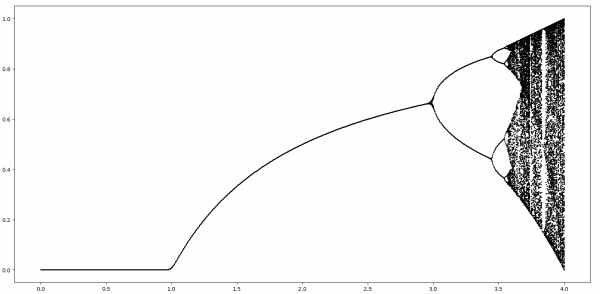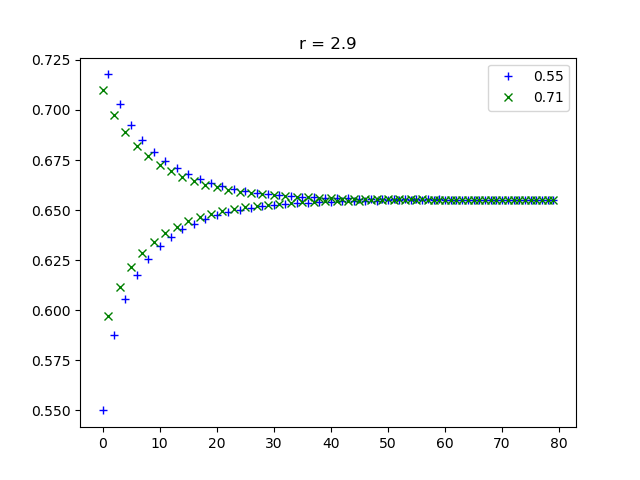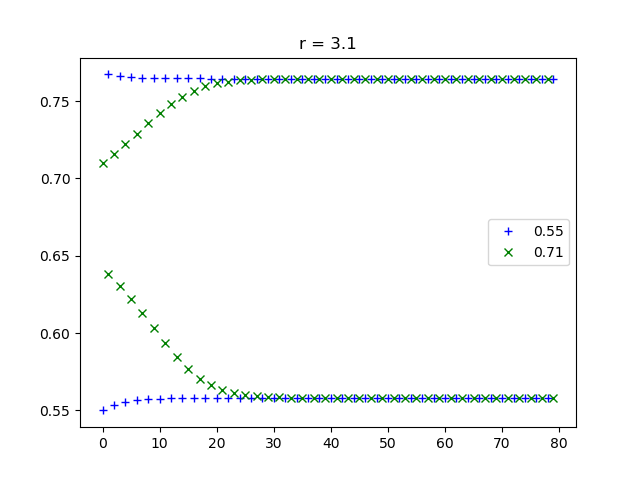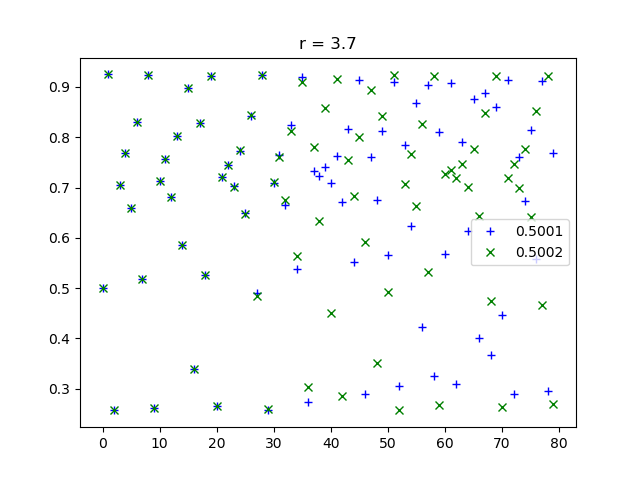# Logistic trajectories

This post is a follow-on to the post on how to make the logistic bifurcation diagram below.That post plotting the attractors for iterations of

f(x) = r x(1 – x).

This post will plot a few trajectories over time for differing starting points and varying values of r.

For values of r less than 3, the iterations converge to the same point regardless of where they start. The fixed point only depends on r. In the plot below, r = 2.9. We start from two different initial points, x = 0.55 and x = 0.71. Both trajectories oscillate toward a common limiting value. (As explained in the earlier post, the limiting value is (r-1)/r = 0.6786.)For values of r a little greater than 3, the iterations oscillate between two values. Here we chose r = 3.1, and again we start from x = 0.55 and x = 0.71.For some larger value of r the iterations rotate between four values, then eight, etc. But for even larger values of r the trajectories are chaotic. Nearby starting points lead to divergent iterations. In the plot below, r = 3.7, which is in the chaotic region. We start with points close to each other, x = 0.5001 and x = 0.5002.Notice how the blue crosses and green x’s are on top of each other for the first 30 iterations or so, then they diverge.

These plots were created using the following Python code.

```import numpy as np
import matplotlib.pyplot as plt

def f(x, r):
return r*x*(1-x)

def time_plot(x0, y0, r, name):
N = 80
x = np.zeros(N)
y = np.zeros(N)
x = x0
y = y0
for i in range(1,N):
x[i] = f(x[i-1], r)
y[i] = f(y[i-1], r)

t = np.arange(N)
plt.plot(t, x, "b+")
plt.plot(t, y, "gx")
plt.legend([str(x0), str(y0)])
plt.title(f"r = {r}")
plt.savefig(name)
plt.close()
```

## 2 thoughts on “Logistic trajectories”

1. nocloud

Thanks for your posts. The value of r mentionned in the text above the first graph should be 2.9 rather than 0.29

2. Thanks.